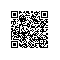# Javascript语法基础

Javascript语法基础

JavaScript中支持数字、字符串和布尔值三种基本数据类型：

1．数字

2．字符串

“”   //空串，它的字符数是0

“hello world!”   //表示字符串hello world

“3.14”

“这个字符串\n共有两行内容”  //其中\n是表示换行的转义字符

“you\'re right,it can\'t be quote” //其中\’是表示单引号的转义字符

3．布尔型

x ＝＝ 2

JavaScript复合数据类型包括对象和数组两种。

1．对象

2．数组

abc

abc

1．数值常量

（1）传统记数法：传统记数法是将一个浮点数分为三个部分，如3.14、10.0。

（2）科学记数法：当一个数字很大或很小的时候，可以使用科学记数法来表示。如1.00E+2、100E-2。

2．字符串常量

3．布尔常量

布尔常量是比较简单的一种常量，只有两种值，一种是true（真），一种是false（假）。在有些时候，JavaScript也可以把0和1分别看成false和true。布尔常量常用于条件判断语句中。

4．null常量

null是空的意思，在JavaScript语言中表示一个“空”的值，一般null主要用于表示完全没有的对象。如果我们希望仅仅声明一个对象存在，并指定它的名称，而暂时不为对象分配内存空间，这时就可以利用null声明一个“空”对象。

注意：在数值数据中null表示0，在字符串数据中null表示一个空串。

1.变量的命名方式

2.变量类型

JavaScript与其他程序语言之间有些区别，其他程序语言大多需要为变量指定一个数据类型，例如将一个变量指定为整数型，那么这个变量就只能存储整数型数据，不可以存储浮点型或其他类型的数据。而JavaScript中的变量是没有类型（notype）的，这就意味着在JavaScript中的变量可以是任何一种数据类型。

3.定义变量

var <标识符> [=<值>]

var PI=3.14;

var tag;

4.定义变量的注意事项

• 变量对大小写敏感（小写y 和大写Y 是两个不同的变量）；
• 变量必须以字母或下划线开始，其后可以是数字或其他字母；
• 变量名不能和javascript中的保留字相同
• 变量最好是先定义后使用

5．变量的值

6．变量的有效范围

7．保留字

1．定义数组

var myArray=new Array()

var mycars=new Array()

mycars="Saab"

mycars="Volvo"

mycars="BMW"

var mycars=new Array(3)

mycars="Saab"

mycars="Volvo"

mycars="BMW"

var mycars=new Array("Saab","Volvo","BMW")

2访问数组

document.write(mycars)

Saab

3修改已有数组中的值

mycars="Opel";

document.write(mycars);

Opel

1．运算符

y=5;

z=2;

x=y+z;

⑴   JavaScript 算术运算符

 运算符 描述 例子 结果 + 加 x=y+2 x=7 - 减 x=y-2 x=3 * 乘 x=y*2 x=10 / 除 x=y/2 x=2.5 % 求余数 (保留整数) x=y%2 x=1 ++ 累加 x=++y x=6 -- 递减 x=--y x=4

⑵   JavaScript 赋值运算符

 运算符 例子 等价于 结果 = x=y x=5 += x+=y x=x+y x=15 -= x-=y x=x-y x=5 *= x*=y x=x*y x=50 /= x/=y x=x/y x=2 %= x%=y x=x%y x=0

⑶  用于字符串的 + 运算符

+ 运算符用于把文本值或字符串变量加起来（连接起来）。如需把两个或多个字符串变量连接起来，请使用 + 运算符。

txt1="What a very";

txt2="nice day";

txt3=txt1+txt2;

txt1="What a very ";

txt2="nice day";

txt3=txt1+txt2;

txt1="What a very";

txt2="nice day";

txt3=txt1+" "+txt2;

⑷ 对字符串和数字进行加法运算

x=5+5;

document.write(x);

x="5"+"5";

document.write(x);

x=5+"5";

document.write(x);

x="5"+5;

document.write(x);

JavaScript 运算符的优先级

 优先顺序 运算符 1 .、（）、[ ] 2 ！、++、typeof、new、void和delete 3 *、/、% 4 +、- 5 <<、>>、>>> 6 <、<=、>、>= 7 = =、!=、= = =、!= = 8 & 9 ^ 10 | 11 && 12 || 13 条件运算符 14 =、+=、-=、*=、/=、%=、<<=、>>=、&=、^= 和 |= 15 ，（注释：此运算符用于将不同的值分开）

2．表达式

（1）常量表达式。常量表达式就是常量本身；

（2）变量表达式。变量表达式就是变量的值；

（3）复合表达式。复合表达式是由常量、变量加上一些运算符所组成的表达式。

10

i+5

（i+10）-sum

JavaScript 保留字

 abstract boolean Break byte case catch char Class const continue extends delete Do double else for function goto if implements import in instanceof int interface long native new null package private protected public return short static super switch synchronized this throw throws transient true try typeof var void while with

JavaScript中的基本数据类型有数字型、字符串型和布尔值三种，除了基本数据类型之外，JavaScript还支持对象、数组、函数、null和undefined数据类型。JavaScript与其他语言一样，也支持常量与变量，不过JavaScript中的变量是无类型的，即可以存储任何一种数据类型的数据。

JavaScript中的运算符比较多，可以分为算术运算符、关系运算符、字符串运算符、赋值运算符、逻辑运算符、逐位运算符和其他运算符七大类。在使用JavaScript中的运算符时，要注意不同运算符的优先次序。在下一章里将会介绍JavaScript中的语句。

本文转自二郎三郎博客园博客，原文链接：http://www.cnblogs.com/haore147/p/4191540.html，如需转载请自行联系原作者使用钉钉扫一扫加入圈子
+ 订阅